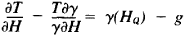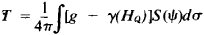# Geodetic Gravimetry

## geodetic gravimetry

[¦jē·ə¦ded·ik grə′vim·ə·trē]
(geodesy)
Worldwide relative measurements of gravitational acceleration used in geodetic studies of the earth.
McGraw-Hill Dictionary of Scientific & Technical Terms, 6E, Copyright © 2003 by The McGraw-Hill Companies, Inc.
The following article is from The Great Soviet Encyclopedia (1979). It might be outdated or ideologically biased.

## Geodetic Gravimetry

a section of geodesy dealing with the theories and methods of using the results of measurement of the force of gravity for solving scientific and practical problems in geodesy. The basic content of geodetic gravimetry is the theories and methods for determining the external field of the potential W of the force of the earth’s gravity g from measurements on the earth’s surface and from astronomical and geodetic materials. It also includes the determination of altitudes and the treatment of astronomical and geodetic grids in connection with the special features of the earth’s gravitational field. It is customary to separate from this field the uniform and known field of potential U of the so-called reference earth, which is represented in the form of a level ellipsoid. The mass centers and the axes of rotation of the real and the normal earth coincide. The fundamental problem of geodetic gravimetry is reduced to the derivation of the perturbing potential T = W - U, which is determined from the solution of the boundary problems of mathematical physics. At the earth’s surface, T satisfies the boundary conditionwhere H is the height over the ellipsoid, γ is the force of gravity in the field U, HQ is the normal height derived from the condition that the increase ∫gdh of the potential W from the start of the gravity head count be measured in the field U, and dh is the incremental excess of the geometric level determination. Several methods involving the solution of the corresponding integral equations were developed for the derivation of T.

In flat regions, some practical problems may be solved using simplified methods for the derivation of T and its derivatives. These methods are based on the condition HQ = 0, which is introduced after calculating the differences g -γ(Hq). This approach, for example, is permissible in astronomical-gravimetric leveling. In this case the problems of geodetic gravimetry will be solved explicitly in closed formulas. The value of T at the earth’s surface is determined by the Stokes formula (1849)where S(ψ) = csc ψ/2 - 3 cos ψ In (sin ψ/2 + sin2 ψ/2) - 6 sin ψ/2 + 1 - 5 cos ψ, ψ = arcsin r/2R, R is the radius of the earth’s sphere, da is its element, and ψ is the arc of a great circle between a fiducial point and a running point with a given force of gravity. This formula describes the external gravitational field of the earth’s sphere. From this equation it is possible to derive the equation for any element of the earth’s gravitational field in its level regions.

Modern geodetic gravimetry is based on the work of M. S. Molodenskii (1945-60) and involves the investigation of methods for the solution of boundary-value problems, conditions of their solubility, and the density and precision of the required measurements.

### REFERENCES

Molodenskii, M. S., M. I. Iurkina, and V. F. Eremeev. “Metody izucheniia vneshnego gravitatsionnogo polia i figury Zemli.” Tr. Tsentral’nogo nauchno-issledovate l’skogo instituta geodesii, aeros’emki i kartografii, 1960, fasc. 131.
Brovar, V. V., V, A. Magnitskii, and B. P. Shimberev. Teoriiafigury Zemli. Moscow, 1961.

M. I. IURKINA